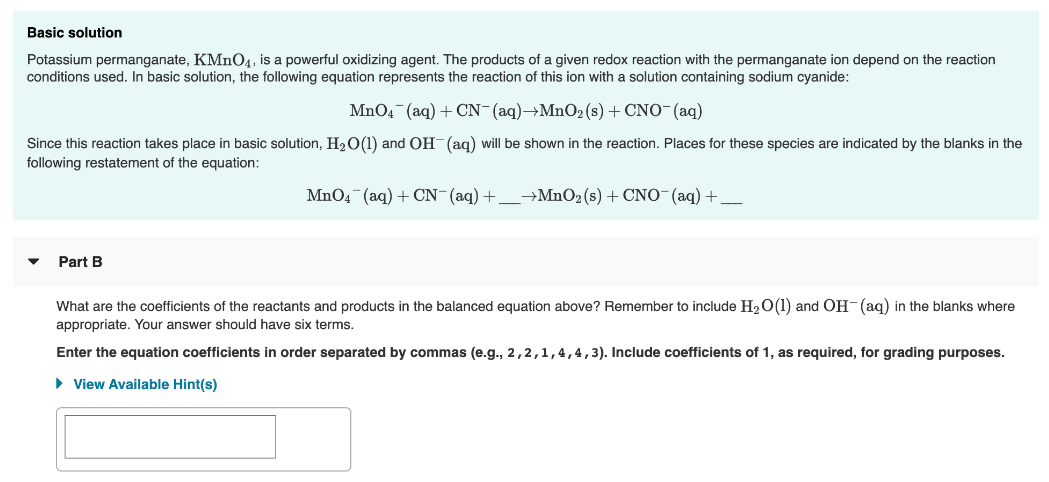# Potassium permanganate, KMnO4, is a powerful oxidizing agent. The products of a given redox reaction with the permanganate ion depend on the reaction condition used. In basic solution, the following equation represents the reaction of this ion with a solution containing sodium cyanide: MnO4^-(aq) + CN^-(aq) → MnO2(s) + CNO^-(aq) Since this reaction takes place in basic solution, H2O(l) and OH^-(aq) will be shown in the reaction. Places for these species are indicated by the blanks in the following restatement of the equation: MnO4^-(aq) + CN^-^-(aq) + ___ → MnO2(s) + CNO^-(aq) ___ What are the coefficients of the reactants and products in the balanced equation above? Remember to include H2O(l) and OH^-(aq) in the blanks where appropriate. Your answer should have six terms. Enter the equation coefficients in order separated by commas (e.g., 2, 2, 1, 4, 4, 3). Include coefficients of 1, as required, for grading purposes.